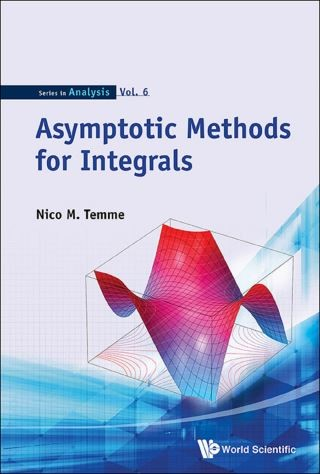## Asymptotic Methods For Integrals• Description
• Author
• Info
• Reviews

### Description

This book gives introductory chapters on the classical basic and standard methods for asymptotic analysis, such as Watson's lemma, Laplace's method, the saddle point and steepest descent methods, stationary phase and Darboux's method. The methods, explained in great detail, will obtain asymptotic approximations of the well-known special functions of mathematical physics and probability theory. After these introductory chapters, the methods of uniform asymptotic analysis are described in which several parameters have influence on typical phenomena: turning points and transition points, coinciding saddle and singularities. In all these examples, the special functions are indicated that describe the peculiar behavior of the integrals.

The text extensively covers the classical methods with an emphasis on how to obtain expansions, and how to use the results for numerical methods, in particular for approximating special functions. In this way, we work with a computational mind: how can we use certain expansions in numerical analysis and in computer programs, how can we compute coefficients, and so on.

Contents:
• Basic Methods for Integrals
• Basic Methods: Examples for Special Functions
• Other Methods for Integrals
• Uniform Methods for Integrals
• Uniform Methods for Laplace-Type Integrals
• Uniform Examples for Special Functions
• A Class of Cumulative Distribution Functions

Readership: Researchers in applied mathematics, engineering, physics, mathematical statistics, probability theory and biology. The introductory parts and examples will be useful for post-graduate students in mathematics.
Key Features:
• The book gives a complete overview of the classical asymptotic methods for integrals
• The many examples give insight in the behavior of the well-known special functions
• The detailed explanations on how to obtain the coefficients in the expansions make the results useful for numerical applications, in particular, for computing special functions
• The many results on asymptotic representations of special functions supplement and extend those in the NIST Handbook of Mathematical Functions